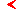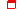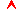﻿ Nonlinear oscillations - Program of course - Education - Saratov group of theoretical nonlinear dynamicsmainthis page only

# Nonlinear oscillations

Authors: Prof. S.P.Kuznetsov and Prof. A.P.Kuznetsov

17 lectureseducationproblems and lessons

Nonlinearity. Oscillations of small and large amplitude, linear and nonlinear. Nonlinearity of the elastic deformations, nonlinearity of springs. Nonlinear capacity, nonlinear inductance and their characteristics. Other examples of nonlinear elements: tunnel diode, electrical discharge etc. Nonlinear oscillating systems.

Weak and strong nonlinearity. Weak nonlinearity as a perturbation of a linear system. N-types of nonlinear characteristics (quadratic, cubic). Strong nonlinearity. Physical examples of systems with weak and strong nonlinearity.

Nonlinear media. Ferromagnetic as an example of a nonlinear medium. Nonlinear media in acoustics and optics. Model nonlinear media.

Isochronism and non-isochronism. Isochronous and non-isochronous oscillations. Examples of the non-isochronism: bouncing ball, pendulum, Kepler’s motion, relativistic electron in magnetic field. Are small oscillations always isochronous?

Non-harmonic oscillations and generation of harmonics. Transformation of the harmonic signals by a nonlinear element with a power-law characteristic. Harmonics and sub-harmonics. Non-harmonic oscillations from spectral and temporal point of view. Physical examples of the nonlinear transformation of spectra: Nonlinearity of the human ear, optical generation of the second harmonic.

Multistability and hysteresis. A concept of multictability: a ball in a potential relief. Examples of multistable systems: mechanical systems, tunnel diode in a circuit with controlled voltage and resistor, ferromagnetic. Hysteresis and its explanation with a model of a ball in a potential relief.

Nonlinear dynamical systems. Representation of equations in a standard form of a set of N differential equations of the first order. Geometrical interpretation og the dynamics: phase space. Examples of dynamical systems of different dimension. Dissipative and conservative systems: a classification based on properties of evolution of a cloud of state points in the phase space.

Conservative nonlinear systems. Examples of conservative systems of the second order. Potential function and the energy integral. Transformation of the mechanical energy under parameter variation for a simple example of mechanical system.

Phase portraits of conservative systems. Strategy of graphical constructing and investigation of a phase portrait of a nonlinear system: A plot of potential energy and its connection with elements of the portrait, fixed points, regions of approximately linear oscillations, separatrix, concept of typical orbits.

Dissipative nonlinear systems and their phase portraits. Introducing dissipation into equation of nonlinear oscillator. Energy as a Lyapunov function, its evolution in time and role in explanation of stability of equilibrium states. Concept of attractor. Basins of attraction. Role of separatrix in a dissipative system as a basin border.

Pendulum equation (Oscillator with sine nonlinearity). A pendulum, a Josephson junction, a problem of deformation of elastic rod (Kirchhoff analogy). Integral of energy and phase portrait. Expression of period of oscillations via elliptic integral. Amplitude dependence of the period. Solution of the pendulum equation associated wit motion on the separatrix. Analysis of the motion in a vicinity of the separatrix. Estimate of a period for the motion near separatrix and spectrum of such motions for the oscillating and rotational solutions.

Oscillator with cubic nonlinearity. Cubic nonlinear oscillator as a paradigm model of the theory of oscillations. Pendulum and other examples. Potential function and phase portraits for two possible signs of the nonlinear term. Asymptotic methods for analytical treatment of oscillations of moderate amplitudes. Secular terms and their excluding. Non-isochronism and non-harmonic character of oscillations of the cubic oscillator: estimate of the correction to the frequency and of amplitude of the third harmonic. Comparison of the approximate results with numerical solution.

Oscillator with quadratic nonlinearity. Quadratic nonlinear oscillator as a paradigm model of the theory of oscillations. Physical examples. Potential function and phase portrait. Asymptotic method and appearance of zero and second harmonics in spectrum. Non-isochronism in the second order of the perturbation theory.

Method of slow complex amplitudes. Application of the slow amplitude approach for dissipative cubic nonlinear oscillator. Definition of the complex amplitude, averaging procedure, reduced equations, and their solution. Conditions of validity of the slow amplitude approach.

Fast and slow motions. Analysis of dynamics of the cubic oscillator with strong dissipation after an initial kick. Reducing to an equation with a small parameter at the highest derivative, and a paradox of insufficient number of initial conditions arising if one attempts to neglect this term. More accurate analysis: distinguishing stages of fast and slow motion, estimate of their duration. Equations for the fast and slow stages and their solution. Sewing together the fast and slow solutions. Depicting fast and slow solutions in the phase portrait.

Self-oscillations and self-oscillators. Examples of self-oscillating systems of different physical nature. The basic features of the self-oscillations. Limit cycle as a mathematical image of the self-oscillations. Self-oscillator as a dissipative system and a limit cycle as an attractor.

Van der Pol and Rayleigh equations. Electronic self-oscillator and its description in terms of the Van der Pol equation. Mechanical self-oscillator and its description in terms of Rayleigh equation. A link of the Van der Pol and Rayleigh equations. Computer solution of the Van der Pol equation: dynamical variable evolution in time, phase portraits. Decaying, quasi-harmonic and relaxation oscillations.

Andronov-Hopf bifurcation and quasi-harmonic self-oscillations. Analysis of the Van der Pol equations in terms of the energy balance equations and using slow amplitude approach. An amplitude Van der Pol equation and its solution. Andronov-Hopf bifurcation and its description in terms of the amplitude equation. Generalized equation for the Andronov-Hopf bifurcation. Normal (supercritical) and backward (subcritical) Andronov-Hopf bifurcation and their bifurcational diagrams.

Relaxation self-oscillations. Rayleigh equation at large control parameter. A variable change to the form with small parameter at the highest derivative. Distinguishing fast and slow motions. Representation of them on the phase plane. Form of the relaxation oscillations in the Rayleigh equation and estimate of their period.

Hard excitation of self-oscillations. Model equation for self-oscillator with hard excitation. Analysis in terms of the energy balance. Slow amplitude method in application to the model equation. Stable and unstable limit cycles. Phase portrait and its evolution under parameter variation. Bifurcations in the system.

Poincare cross-section construction. The main idea of the Poincare cross-section construction. The Poincare map obtained numerically and via the slow-amplitude analysis for the Van der Pol oscillator and for self-oscillator with hard excitation. Some simple basic properties of the one-dimensional iterative maps: fixed points and their stability. Iteration diagrams.

Nonlinear resonance. Periodically forced nonlinear oscillator as an example of three-dimensional dynamical system. Phase space on the cylinder. Analysis of the forced nonlinear oscillator in terms of the slow amplitudes. Bistability and hysteresis associated with the nonlinear resonance. Cusp point and fold curves in the parameter plane of amplitude and frequency of the external force. Nonlinear resonance on harmonics and sub-harmonics. A possibility of complex oscillation regimes: the dynamical chaos.

Synchronization. Amplitude equation for the periodically forced Van der Pol oscillator. Approximation corresponding to motion at the unperturbed limit cycle (the Adler equation). Periodic and quasiperiodic regimes of the forced self-oscillator. Arnold tongue as a domain of syncronization in the parameter plane. Phase portrait of the amplitude equation and its analysis. Two basic mechanisms of breack-up of synchronization. Cusp point and fold curves on the parameter plane, Andronov-Hopf bifurcation at large amplitudes of forcing.  Evolution of spectra of the forced self-oscillator in dependence of parameters at small and large amplitude of the external force.maineducationthis page onlytop

Saratov group
of theoretical nonlinear
dynamics